Chapter Notes: Numbers from One to Nine

# Chapter Notes: Numbers from One to Nine - Notes | Study Mathematics for Class 1: NCERT - Class 1

 Table of contentsCan You Count the Number of Toffees?What is As Many As?What is More or Less?What are Missing Numbers?1 Crore+ students have signed up on EduRev. Have you?

Can You Count the Number of Toffees?

Let us count the number of toffees given below and learn how to write the numbers.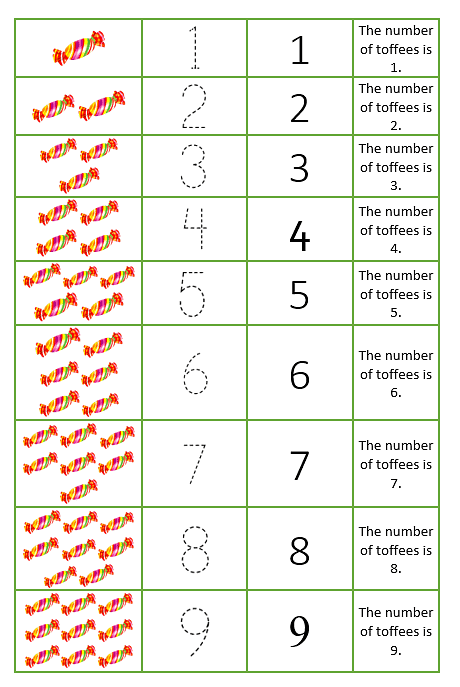What is As Many As?
As many as is all about counting and finding out that the objects present on both sides are equal to each other.There are 3 Icecreams on both sides

Let us understand this concept.
Example: Megha has 4 dolls. She needs as many dresses as she has dolls.
So how many dresses she needs for them?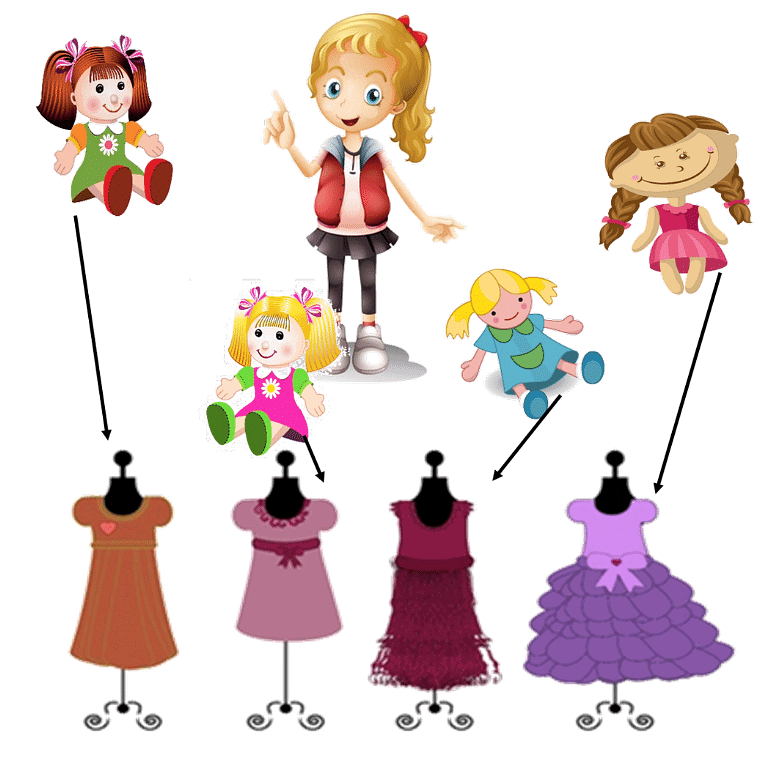Megha needs 4 dresses for 4 dolls.

Question for Chapter Notes: Numbers from One to Nine
Try yourself:You have 5 friends and you want to give 1 chocolate to each of your friends. How many chocolates do you need to buy for 5 friends?What is More or Less?

More means greater, and less means smaller.

• In the numbers 3 and 2, we know that 3 is greater than 2.  This means,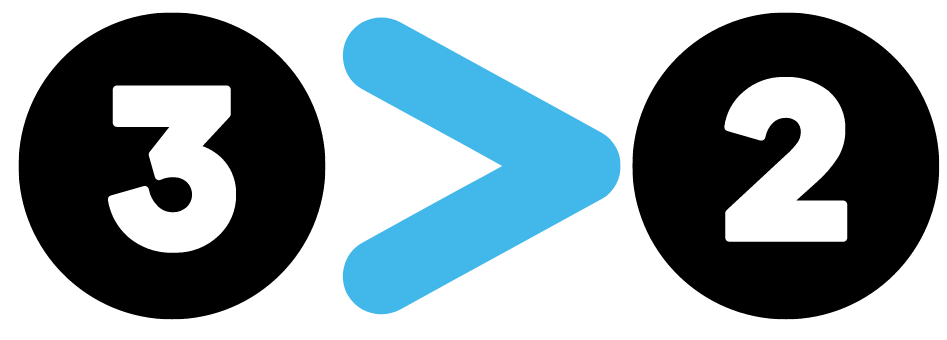• We can also say 2 is less than 3. This means,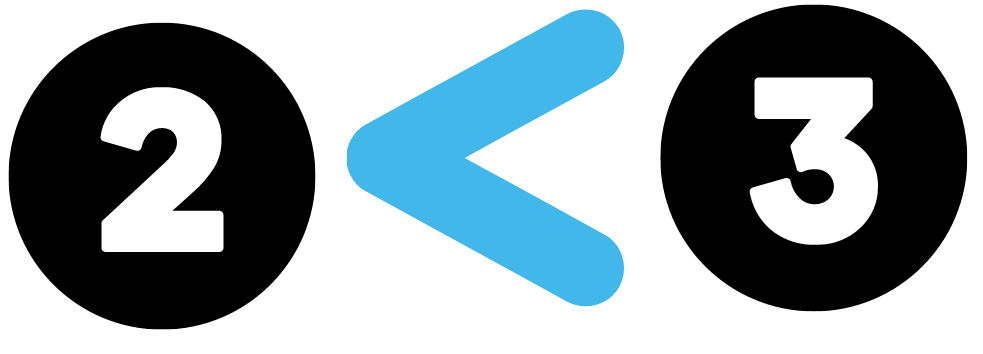Example 1: Rahul and Megha are fond of candies and toys. Let’s count how many candies/toys they have.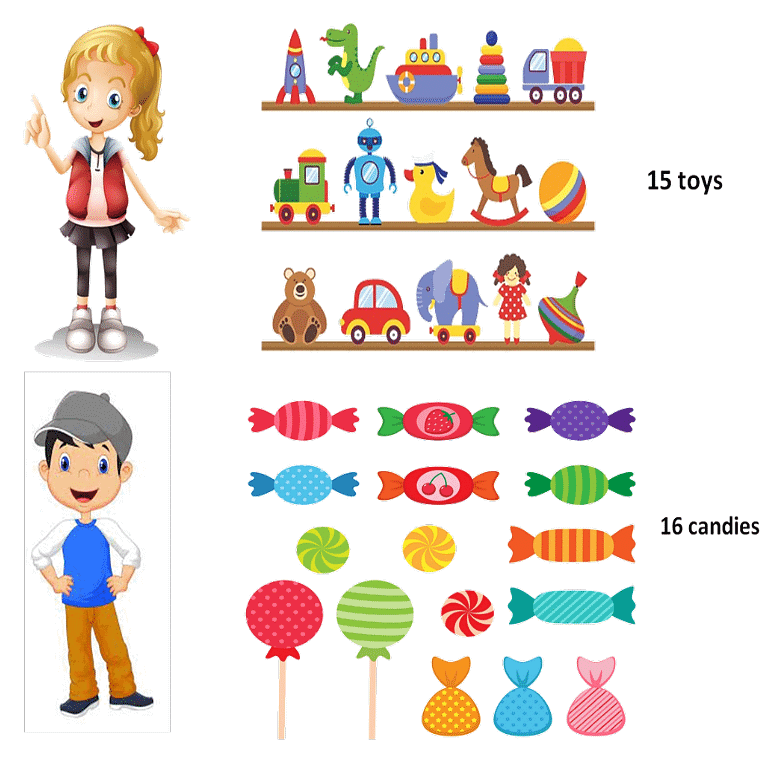Megha has 15 toys

Rahul has 16 candies.

So, Rahul has a greater number of candies.

Example 2:  Look at the picture given below. Can you tell which basket has more fruits?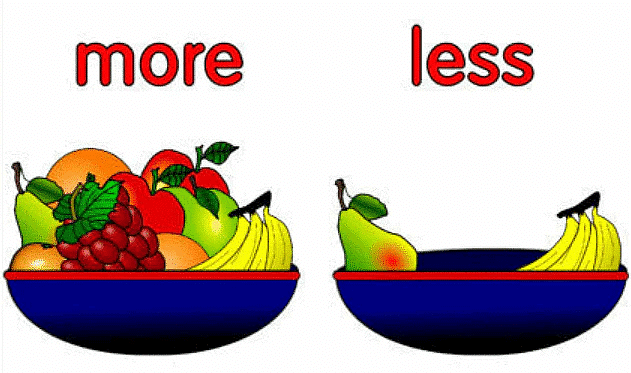The basket on the left has 9 fruits.

The basket on the right has 2 fruits

So, there are more fruits in the basket on the right.

What are Missing Numbers?

A series or sequence where a number is missed is known as a missing number sequence.

Look at the example given below. Numbers given are 1, ___, ___, 4, ___, ___, 7, ___, ___.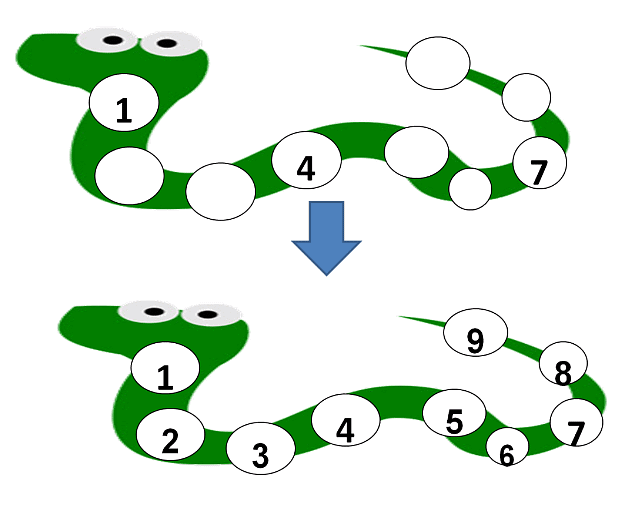We just learned the counting above, so we know that the correct series will be 1, 2, 3, 4, 5, 6, 7, 8, 9.

Question for Chapter Notes: Numbers from One to Nine

2,___, 4,___, 6

What is Zero?

Let's understand the concept of zero with the help of an example.

How many chocolates do you see in the given picture?If you give these 2 chocolates to your best friend, then how many chocolates will be left?

There will be 0 chocolates left.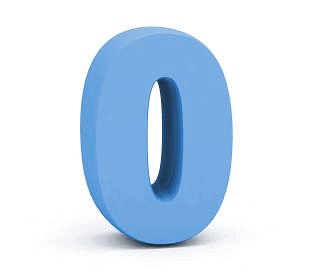Zero

The document Chapter Notes: Numbers from One to Nine - Notes | Study Mathematics for Class 1: NCERT - Class 1 is a part of the Class 1 Course Mathematics for Class 1: NCERT.
All you need of Class 1 at this link: Class 1

## Mathematics for Class 1: NCERT

47 videos|67 docs|25 tests
 Use Code STAYHOME200 and get INR 200 additional OFF

## Mathematics for Class 1: NCERT

47 videos|67 docs|25 tests

Track your progress, build streaks, highlight & save important lessons and more!

,

,

,

,

,

,

,

,

,

,

,

,

,

,

,

,

,

,

,

,

,

;